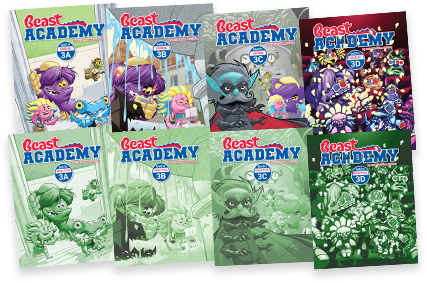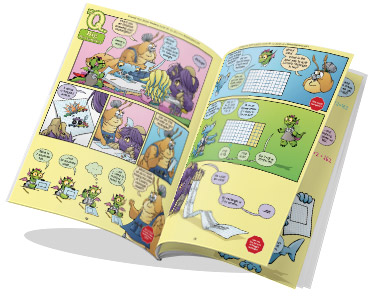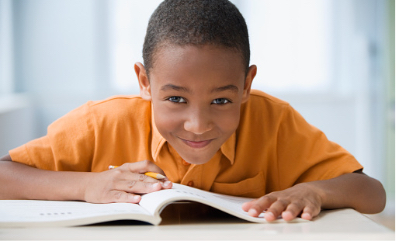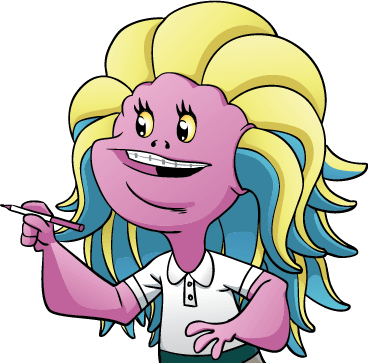By Jason Batterson and Shannon Rogers
Illustrated by Erich Owen

# Level 3 Math Books

Ages 9‑10By Jason Batterson and Shannon Rogers
Illustrated by Erich Owen\$118.00

## Level 3 Books Information

Designed in an engaging comic-book style format, our Level 3 curriculum includes the Guide books and Practice books for all four units (3A, 3B, 3C, 3D).

• The Guide book is a full-color paperback textbook, which teaches concepts in a comic-book style.
• The Practice book complements the Guide book and includes 300+ problems ranging from introductory-level exercises to challenging puzzles and word problems.Level 3 has four units: A, B, C and D. Topics build upon each other from unit to unit.

3A
3B
3C
3D
Shapes
Angles, triangles, quadrilaterals, other polygons, puzzles with polyominoes.
Multiplication
Times tables, the commutative and associative properties, multiplying numbers that end in one or more zeroes.
Variables
Writing expressions and equations, evaluating expressions, solving equations.
Fractions
Unit fractions, fractions on the number line, mixed numbers, equivalent fractions, parts of a whole, comparing and ordering fractions, simplifying fractions.
Skip-Counting
Patterns with repeated addition, arithmetic sequences, foundations for understanding multiplication, the distributive property, and factoring.
Perfect Squares
Properties of squares, squaring numbers that end in 5, finding the next perfect square, multiplying nearby numbers using perfect squares.
Division
Division models and strategies, relating multiplication and division, finding a quotient and remainder.
Estimation
Rounding, computing estimates, over- and underestimating, eyeball estimates, Fermi problems.
Perimeter & Area
Perimeter and area of rectangles, polygons, and rectilinear shapes.
The Distributive Property
Order of operations, area models of the distributive property, multiplying multi-digit numbers.
Measurement
Length, weight, volume, temperature, money, and time. Metric and customary units.
Area
Square units, adding and subtracting areas, tangrams, triangle area, unit conversions.

We recommend taking the Level 3A Placement Test to determine if your student is ready to take Beast Academy Level 3.

Solutions to the placement test are found at the end of the test. Your student may also take the Level 3B, 3C, or 3D placement tests to determine readiness per unit.Get the guide books for sure, because they are endearing and interesting and adorable and wonderful and they make your kid fall in love with doing math (even if they already love math).

Kjersti, BA parent